## Tuesday, September 30, 2008

### 136

136 = 23 x 17.

136 is the smallest number (except 1) such that the sum of the cubes of the digits of the sum of the cubes of its digits equals itself: 13 + 33 + 63 = 244 and 23 + 43 + 43 = 136.

136 is a refactorable number (divisible by the count of its divisors); it has a total of 8 divisors (1, 2, 4, 8, 17, 34, 68, 136), with 8 among them.

136 is a triangular number, which can be represented in the form of a triangular grid of points, where the first row contains a single element and each subsequent row contains one more element than the previous one. Hence, it is the sum of the first 16 positive integers.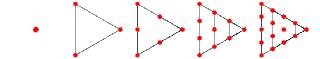A representation of the first few triangular numbers.
136 is 10001000 in base 2 (binary) and 88 in base 16.
136 has a representation as a sum of two squares: 136 = 62 + 102.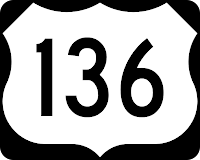136 Austria is an asteroid discovered in 1874.

## Monday, September 29, 2008

### 125

125 is a perfect cube, 53.

125 is the smallest cube that is also the sum of two squares in two different ways: 125 = 112 + 22 = 102 + 52.

5
3 – 27 = 5 – 2. The only known matching pattern is 133 – 37 = 13 – 3.

125 is the hypotenuse of a primitive Pythagorean triple: 1252 = 442 + 1172.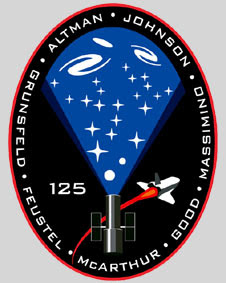Space Shuttle mission
STS-125 was the final servicing mission to the Hubble Space Telescope.

Source: The Penguin Dictionary of Curious and Interesting Numbers, rev. ed., by David Wells.

## Friday, September 26, 2008

### 19600

19600 = 1402, a perfect square.

19600 = 24 x 52 x 72.

19600
is one of only three numbers that are simultaneously square and tetrahedral. Tetrahedral numbers correspond to the number of balls in a triangular pyramid in which each side contains n + 1 balls. They are the sum of consecutive triangular numbers. 19600 is the 48th tetrahedral number.

19600 =
1402
= 1 + 3 + 6 + 10 + 15 + . . . + 1176.

The others are 1 = 1
2 = 1 and 4 = 22 = 1 + 3.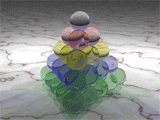A triangular pyramid with side length 5 contains 35 spheres. Each layer represents one of the first five triangular numbers.

19600 has a representation as a sum of two squares: 19600 = 842 + 1122.A contestant in Nathan’s Famous Hot Dog Eating Contest in New York who consumes 66 hot dogs (the world record) would ingest a total of 19,600 calories.

Sources:
Wolfram MathWorld; Wikipedia; CalorieLab

## Thursday, September 25, 2008

### 6174

6174 = 2 x 32 x 73.

6174 has four 4s in base 5: 144144.

6174
is Kaprekar’s Constant, named for D.R. Kaprekar of Devlali, India.

Choose a four-digit number, as long as the four digits are not all identical. Rearrange the four digits to get the largest and smallest numbers these digits can make. Subtract the smallest number from the largest to get a new number. Repeat these operations for each new number. For any four-digit number, the process produces 6174 in at most seven steps, and the calculation repeats.

5200 – 0025 = 5175
7551 – 1557 = 5994
9954 – 4599 = 5355
5553 – 3555 = 1998
9981 – 1899 = 8082
8820 – 0288 = 8532
8532 – 2358 = 6174
7641 – 1467 = 6174

6174 is also a Harshad (or Niven) number, which is divisible by the sum of its digits.

On the standard 33-hole cross-shaped peg solitaire board, 6174 is the number of distinct board positions after 9 jumps that can still be reduced to one peg at the center, starting with the center vacant.

The square root of the sums of the squares of the prime factors of 6174 is a prime: 22 + 32 + 32 + 72 + 72 + 72 = 169 = 132, and 13 is a prime.Source: Mudd Math Fun Facts; Wolfram MathWorld; Plus

## Wednesday, September 24, 2008

### 157

157 is a prime number.

157
is the smallest prime number of the form 2p + p3, where p is prime.

157 is the smallest three-digit prime that produces five other primes by changing only its first digit: 257, 457, 557, 757, and 857.

1572 and (157 + 1)2 use the same digits: 24649 and 24964.The most common type of
Escherichia coli infection that causes illness in people is E. coli 0157.Source:
Prime Curios!
Image: CDC/Peggy S. Hayes

## Tuesday, September 23, 2008

### 71

71 is the 20th prime number.

The numbers 2, 5,
71, 369119, and 415074643 are the only known numbers that divide the sum of all the primes less than themselves.

71 is a centered heptagonal number: 1, 8, 22, 43, 71, 106, 148, 197, . . .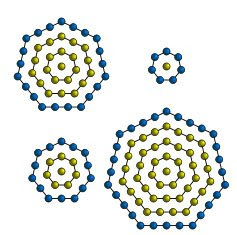71 is a Google prime. The nth Google number is the first n-digit prime found in the decimal expansion of e: 2, 71, 271, 4523, 74713, . . . The name stems from a famous job ad created by Google that said: “{first 10-digit prime found in consecutive digits of e}.com.”
Some insinuated that each letter could influence the following one and that the value of MCV in the third line of page 71 was not the one the same series may have in another position on another page, but this vague thesis did not prevail.—Jorge Luis Borges, “The Library of Babel”

Source:
Number Gossip; Wikipedia
Image: Wikipedia (Claudio Rocchini)

## Monday, September 22, 2008

### 23

23 is the ninth prime number, the smallest odd prime that is not a twin prime.

23 is one of only two integers that needs nine (positive) cubes to represent it: 23 = 23 + 23 + 13 + 13 + 13 + 13 + 13 + 13 + 13.

There were
23 problems on David Hilbert’s list of unsolved mathematical problems, presented to the International Congress of Mathematicians in Paris in 1900.

For
23 or more people in a room, the probability that two people have the same birthday is greater than 50%.

23! is 23 digits long.The speed limit on campus at Tulane University.

Sources:
Number Gossip; Wikipedia
Photo: M. Pearson

## Friday, September 19, 2008

### 529

529 = 232. It is a perfect square.

529 is the 32rd lazy caterer number: the maximum number of pieces in which it is possible to divide a circle (pancake or pizza) for 32 cuts.

The concatenation of the consecutive numbers 528 and 529 forms a square number: 528529 = 7272.Pancake cut into seven pieces with three straight cuts.

When it comes to genuine computation, however, to seeing a self-important number like 529 and panicking when you divide it into 2,200, or realizing that, hey, it's the square of 23! well, that calls for a very different number system, one that is specific, symbolic and highly abstract.—Natalie Angier, New York Times, Sept. 15, 2008.

Section 529 of the IRS tax code organizes 529 plans to encourage saving for higher education.

## Thursday, September 18, 2008

### 11 185 272

11 185 272 is the number of decimal digits in the 46th known Mersenne prime, discovered on Sept. 6, 2008, by Hans-Michael Elvenich in Germany, a participant in the Great Internet Mersenne Prime Search.

A Mersenne prime has the form 2
p – 1, where p is a prime number. The 46th Mersenne prime, the second largest identified to date, is 237156667 – 1. A larger Mersenne prime, 243112609 – 1, was discovered on Aug. 23, 2008. The 46th Mersenne prime is the first prime to be discovered out of order since Walt Colquitt and Luther Welsh Jr. found 2110503 – 1 (the 29th Mersenne prime) in 1988.

Source:
Great Internet Mersenne Prime Search; Mersenne Primes

## Wednesday, September 17, 2008

### 54

54 = 2 x 33.

54
is the smallest number that can be written as a sum of three squares in three ways: 54 = 12 + 22 + 72 = 22 + 52 + 52 = 32 + 32 + 62.

54 is the number of integer partitions of 19 into distinct parts.

A polygonal number can be represented as nested points arranged in the form of a regular polygon. 54 is a nonadecagonal (19-gonal) number: 1, 19, 54, 106, 175, . . .

54 is 110110 in base 2 and 66 in base 8.Sources:
Wikipedia; Number Gossip

## Tuesday, September 16, 2008

### 12 978 189

12978189 = 32 x 72 x 29429.

12978189 has a representation as a sum of two squares: 12978189 = 4832 + 35702.

12 978 189 is the number of decimal digits in the world’s largest known prime number, discovered on a UCLA computer on Aug. 23, 2008, as part of a 12-year-old, worldwide computing project called the Great Internet Mersenne Prime Search (GIMPS).

This number is the 45th known Mersenne prime, 2
43112609 – 1.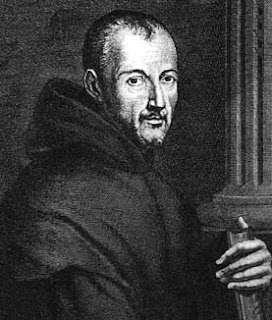Marin Mersenne (1588–1648)

Source:
Great Internet Mersenne Prime Search.
Image: Courtesy of
Loci: Convergence

## Monday, September 15, 2008

### 180

180 = 22 x 32 x 5.

1803 is the sum of consecutive cubes: 1803 = 63 + 73 + 83 + . . . + 683 + 693.

180 has a representation as a sum of two squares: 180 = 62 + 122.

In the plane, the interior angles of a triangle add up to 180 degrees.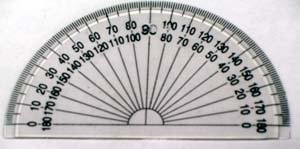The number of Fahrenheit degrees between the freezing point of water, 32, and its boiling point, 212, is 180.

Source: Wells, D. 1997.
The Penguin Dictionary of Curious and Interesting Numbers, rev. ed.

## Friday, September 12, 2008

### 1529

1529 = 11 x 139.

The square of 1529 is the sum of two or more consecutive squares: 15292 = 2337841.

1529 is the number of primitive subsequences of {1, 2, 3, . . . 16, 17}.

1529 is the sum of a positive square and a positive cube in more than one way.

1529 is the 11th term in a sequence defined by the following bilinear recurrence:
Sn+4 Sn = Sn+3 Sn+1 + S2n+2 (n = 1, 2, . . . ) with the initial condition S1 = S2= S3= S4= 1.

This sequence begins 1, 1, 1, 1, 2, 3, 7, 23, 59, 314,
1529, 8209, 833313, 620297, 7869898, . . . .

Interestingly, all the terms are integers even though calculating
Sn+4 a priori involves dividing by Sn. This sequence was discovered by Michael Somos and is associated with the arithmetic of elliptic curves.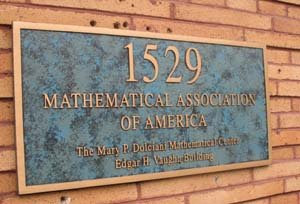1529 18th Street, N.W., is the address of the Mathematical Association of America in Washington, D.C.

Source: Everest, E., S. Stevens, D. Tamsett, and T. Ward. Preprint (Feb. 1, 2008). Primes generated by recurrence sequences.

## Thursday, September 11, 2008

### 911

911 is a prime number. It is a Sophie Germain prime because 2 x 911 + 1 = 1823 is a prime.

911 is both deficient and odious. The sum of all its positive divisors except itself is less than 911 (deficient), and its binary expansion has an odd number of 1s (odious).

Each term of a Fibonacci-like sequence is the sum of the previous two terms. If you start with 9 and 11, the 11th term in the sequence is 911.

911 is the sum of three consecutive primes: 911 = 293 + 307 + 311.

911 is a number that cannot be written as a sum of three squares.911 is the emergency telephone number in the United States.

## Wednesday, September 10, 2008

### 20

20 = 22 x 5.

20 is the smallest number that cannot be prefixed or followed by one digit to form a prime.

20 has a representation as a sum of two squares: 20 = 42 + 22.

20 is the fourth tetrahedral number.

20 is 22 in base 9.

An icosahedron is a polyhedron with 20 triangular faces. The prefix icos comes from the Greek word for “twenty.”Source: Number Gossip